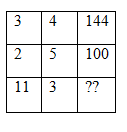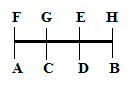# SSC CGL 2018 Practice Test Papers | Reasoning Ability (Day-48)

Dear Aspirants, Here we have given the Important SSC CGL Exam 2018 Practice Test Papers. Candidates those who are preparing for SSC CGL 2018 can practice these questions to get more confidence to Crack SSC CGL 2018 Examination.

[WpProQuiz 3420]

Click “Start Quiz” to attend these Questions and view Explanation

1)  If \$ means ‘added to’, # means ‘multiplied by’, % means ‘divided by’ and & means ‘difference of’ then what is the value of  8 \$ 40 % 8 # 7 & 20.

a) 9

b) 20

c) 25

d) 23

2) From the given alternatives select the word which cannot be formed using the letters of the given word.

GLOBALISATION

a) BALL

b) SALINE

c) LOG

d) BAG

3) Find the missing letter in the given table.a) 1090

b) 1089

c) 1296

d) 1156

4) Unscramble these letters to make a ______.

FPCIIAC

a) City

b) Pacific

c) Mountain

d) Country

Eight persons are sitting in two parallel rows of four seats each. A,B, C and D  are sitting in row II facing north and E, F, G and H are sitting in row I facing south. G is facing neighbor of A. B sits at one end of the row. G and E are immediate neighbors. D is neighbor of both B and C. H faces B and E faces immediate neighbor of B. G sits to the right of E.

5) Who sits to the immediate right of H?

a) E

b) G

c) F

d) C

6) Find the odd one.

a) C

b) H

c) F

d) A

7) What is the position of A with respect to B?

a) Immediate left

b) Immediate right

c) Third to the left

d) Second to the right

8) If A is related to G, C is related to E then D is related to which of the following?

a) F

b) H

c) D

d) None of the above

9) After changing every letter to next letter of the alphabetic series then which of the following word has 2 vowels?

a) BCDH

b) RHIP

c) QERT

d) DHYA

10) Select the related word/ number from the given alternatives.

20: 30 :: 40:?

a) 70

b) 50

c) 60

d) 90

8 \$ 40 % 8 # 7 & 20

=> 8+40÷8×7-20

=8+5×7-20

=8+35-20=23

Word SALINE cannot be formed since E is not there in the given word.

3×4=12; 122=144

2×5=10; 102=100

11×3=33; 332=1089

PACIFIC

Direction (5 – 8):Except C all others are sitting at ends of the rows.

DHYA — After changing to next letter => EIZB

20 ×2 – 10 = 40 – 10 = 30

40× 2 – 10 = 80 – 10 = 70